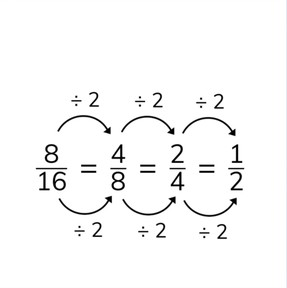Simplifying fractions

# Simplifying fractions

I can reduce simple fractions.

No account needed.8,000 schools use Gynzy92,000 teachers use Gynzy1,600,000 students use Gynzy

## General

Fractions can be intimidating to students who have just become comfortable working with whole numbers. But by explaining fractions through real-life visuals and examples such as pizza or pie, students can better understand how fractions with different numerators and denominators can be equivalent to one another. This lesson will also teach students how to divide fractions by the greatest common factor in order to properly simplify them.

4.NF.A.1

## Learning Objective

Students will be able to reduce simple fractions.

## Introduction

Students will match fractions to colored bars. They will also match fractions to partly-eaten pizzas.

## Instruction

The process to reduce fractions will be explained. Students will learn how to divide the numerator and denominator by the same number (the GCF). Students will practice reducing fractions with different visuals, like colored bars and pizzas, and finding the greatest common denominators. They will also reduce fractions with a larger numerator than denominator.

## Quiz

Review reducing fractions with 6 multiple-choice and 4 short answer questions.

## Closing

Reflect on what students have learned about reducing fractions and practice reducing some more fractions.

## Other fraction resources

We offer multiple fraction lessons and tools to help your students learn about fractions in a way that is engaging and accessible. Two essential tools are our fraction bar and fraction circle widgets, which can be easily customized and give students a visual reference for understanding fractions.

We also have two very fun fraction games which give students a fun way to practice recognizing fractions. Our fraction flag game has users select the denominator they would like to work with. They are then shown a grid that they then have to color in based on the instructions given. For example, if you choose 1/4 as your fraction, you will be shown multiple 4x4 "flags," and then asked to color in a certain percentage of the flag different colors. For example, fill 2/4ths of the flag in with blue, and then 1/4th of the flag in both yellow and green. In this way, students have a fun way of practicing their fractions.

Our other fraction game is called Bubble: Fractions, in which students must navigate their octopus to "eat" the fraction displayed on the screen.

### The online teaching platform for interactive whiteboards and displays in schools

• Save time building lessons

• Manage the classroom more efficiently

• Increase student engagement